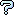All about flooble | fun stuff | Get a free chatterbox | Free JavaScript | Avatarsperplexus dot infoSo close, but no closer... (Posted on 2014-12-04)Determine the value of the smallest positive integer N such that N1/N contains precisely one zero immediately following the decimal point.

Too easy?

How about seven zeroes, then?

 Submitted by broll Rating: 3.0000 (2 votes) Solution: (Hide) In general, the mth qualifying number (counting 38 as the zeroeth such number) of the form (n-1)^(1/(n-1)) has the form (as string): "1.(m zeroes)1(m+2 zeroes)1"; since the last part is so small it can be considered as 1; 1.1001, 1.010001, 1.00100001, 1.0001000001, etc. This is the form 1+1/(10^(m+1))+1/(10^(2m+4)). So the function (1+1/(10^(m+1))+1/(10^(2m+4)))^(N-1)+1 = N returns n as the integer part of N. When m=5, N=16626518.569717461698..., so n = 16626518. When m=6, N=190660035.08011929935..., so n = 190660035. When m=7, N= 2148818406.4441059949..., so n= 2148818406. and so on.Comments: ( You must be logged in to post comments.)
 Subject Author DateEasy part Math Man 2014-12-08 07:43:32re(3): computer assisted solution, a question broll 2014-12-05 00:37:57re(2): computer assisted solution,,,a question Ady TZIDON 2014-12-05 00:18:20re: computer assisted solution broll 2014-12-04 23:19:46computer assisted solution Charlie 2014-12-04 14:21:37Please log in:

 Search: Search body:
Forums (0)